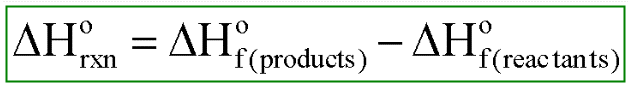# Problem: Calculate ΔH° for each of the following reactions, which occur in the atmosphere.c. SO3 (g) + H2O (l) → H2SO4 (aq)

###### FREE Expert Solution

The enthalpy of a reaction can be determined if we are given the enthalpy of formation value for each compound89% (118 ratings)###### Problem Details

Calculate ΔH° for each of the following reactions, which occur in the atmosphere.

c. SO3 (g) + H2O (l) → H2SO4 (aq)